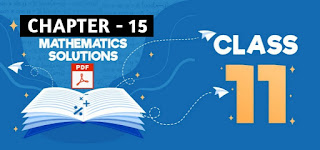# Limits and Continuity: Class 11 Mathematics Solutions | Exercise - 15.1## Chapter - 15  Limits and Continuity

In this chapter, we are basically going to learn about the methods of finding Limits of normal function and trigonometric functions. We will also learn to identify whether the given function is continuous or discontinuous at x = a.

So before moving towards the solution section, let us have a little concept about the subtopics involved in limits and continuity.

### Introduction

We have discussed a function and its graph in chapter I. As a review, we give the definition of a function, its domain, range and its graph once again before defining the limit of a function.

We deal with limits and continuity which are quite fundamental for the development of calculus. These two concepts are closely linked together with the involvement of the concept of limit in the definition of continuity. So in the sequence, limit comes first and it is proper to begin with some discussion about it.

The discussion is initiated with some examples so as to give some intuitive idea about it. Then follows the precise definition of the limit. The same line of approach is being followed in the case of continuity as well. We shall also mention some limit theorems and properties of continuous functions without proof.

### Function

Let X and Y be two non-empty sets. Then a function f from X to Y is a rule which assigns a unique element of Y to each element of X. The unique element of Y which ƒ assigns corresponding to an element x ∈ X is denoted by f(x).

So, we also write y = f(x) The symbol f : X→Y usually means 'f is a function from X to Y'. The element f(x) of Y is called the image of x under the function f.

### Value of the Function

If f is a function from X to Y and x = a is an element in the domain of f, then the image f(a) corresponding to x = a is said to be the value of the function at x = a.

If the value of the function f(x) at x = a denoted by f(a) is a finite number, then f(x) exists or is defined at x = a otherwise, f(x) does not exist or is not defined at x = a.

For example:
i. y = f(x) = 3x + 5 exists or is defined at x = 2as
f(2) = 3 * 2 + 5 = 11 is a finite number

ii. y = f(x) = 1/(x - 1) is not defined at x = 1 as
f(1) = 1/0 is not a finite number.
Hence f(x) does not exist or is undefined at x = 1.

### Exercise - 15.1

This PDF contains all the solutions of class 11, Limits and Continuity Chapter. It only contains solutions of exercise - 15.1. If you want solutions of exercise - 15.2 and exercise -  15.3 then you'll find them above. You just need to click on the button given above.

NoteScroll the PDF to view all Solution

You are not allowed to post this PDF in any website or social platform without permission.

## Is Class 11 Mathematics Guide Helpful For Student ?

I have published this Notes for helping students who can't solve difficult maths problems. Student should not fully depend on this note for completing all the exercises. If you totally depend on this note and simply copy as it is then it may affect your study.

Student should also use their own will power and try to solve problems themselves. You can use this mathematics guide PDF as a reference. You should check all the answers before copying because all the answers may not be correct. There may be some minor mistakes in the note, please consider those mistakes.

## How to secure good marks in Mathematics ?

As, you may know I'm also a student. Being a student is not so easy. You have to study different subjects simultaneously. From my point of view most of the student are weak in mathematics. You can take me as an example, I am also weak in mathematics. I also face problems while solving mathematics questions .

If you want to secure good marks in mathematics then you should practise them everyday. You should once revise all the exercise which are already taught in class. When you are solving maths problems, start from easy questions that you know already. If you do so then you won't get bored.

Maths is not only about practising, especially in grade 11 you to have the basic concept of the problem. When you get the main concept of the problem then you can easily any problems in which similar concept are applied.

When your teacher tries to make the concept clear by giving examples then all students tries to remember the same example but you should never do that. You can create your own formula which you won't forget later.

If you give proper time for your practise with proper technique then you can definitely score a good marks in your examination.

Disclaimer: This website is made for educational purposes. If you find any content that belongs to you then contact us through the contact form. We will remove that content from our as soon as possible.

If you have any queries then feel free to comment down.
No Comment